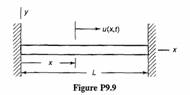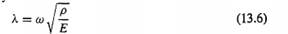### Create an Account

Home / Questions / The uniform bar shown in Fig P99 has uniform cross sectional area A modulus of elasticity ...

# The uniform bar shown in Fig P99 has uniform cross sectional area A modulus of elasticity E and mass density p It is attached to rigid supports at its ends a Obtain the characteristic equation

The uniform bar shown in Fig. P9.9 has uniform cross-sectional area A, modulus of elasticity E, and mass density p. It is attached to rigid supports at its ends, (a) Obtain the characteristic equation from which eigenvalues for axial free vibration can be determined. Your answer should contain the parameters λ and L, where X is defined by Eq. 13.6. (b) Determine an expression for the fundamental axial frequency of the bar (ω rad/s). Your answer should contain the parameters λ, p, and L. (c) Determine an expression for the fundamental axial mode shape, φi(x), and use M a t l a b , or another computer program, to plot the fundamental mode. Scale the mode so that.its maximum value is l.CLJun 30 2020 View more View LessSubscribe To Get Solution